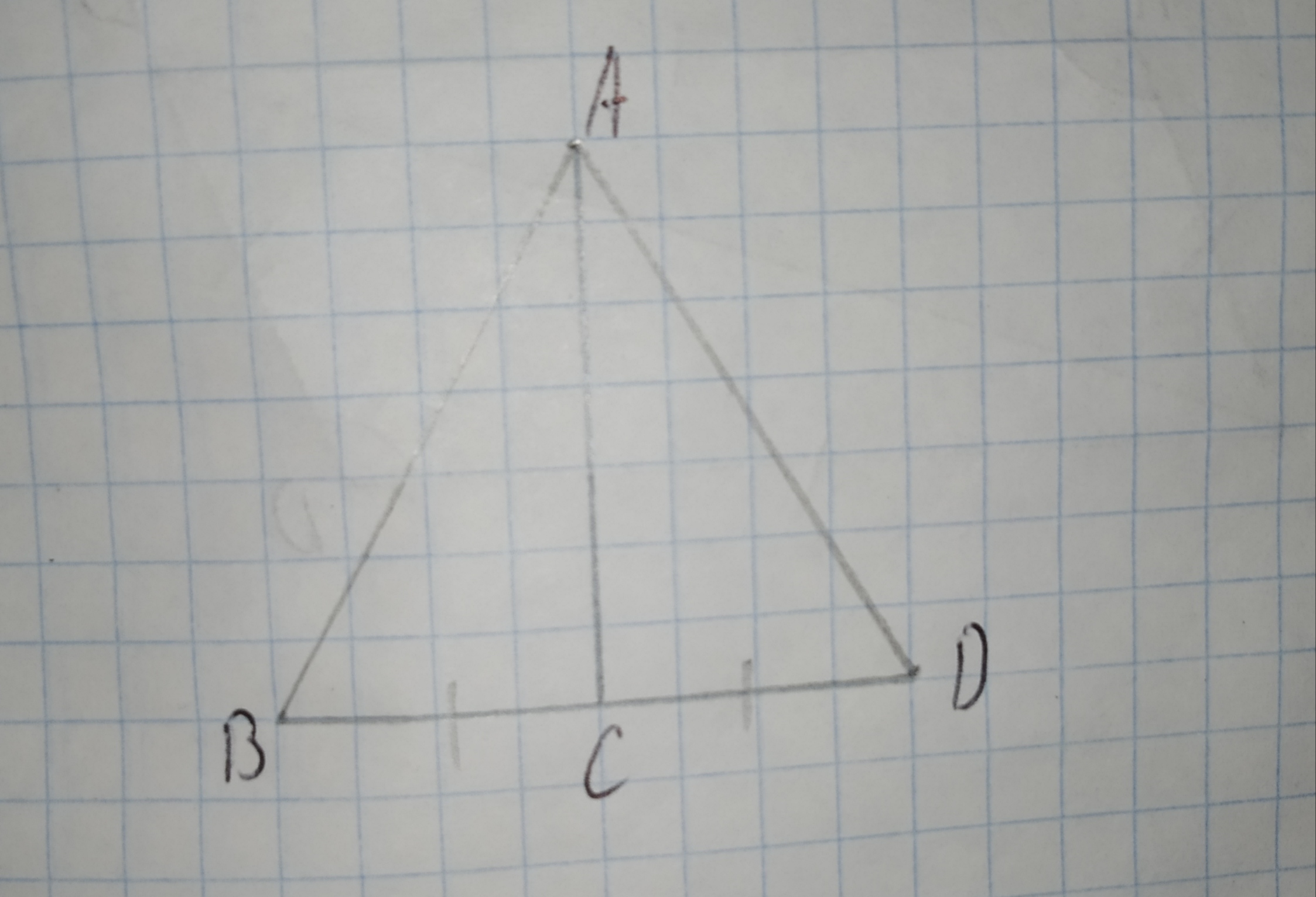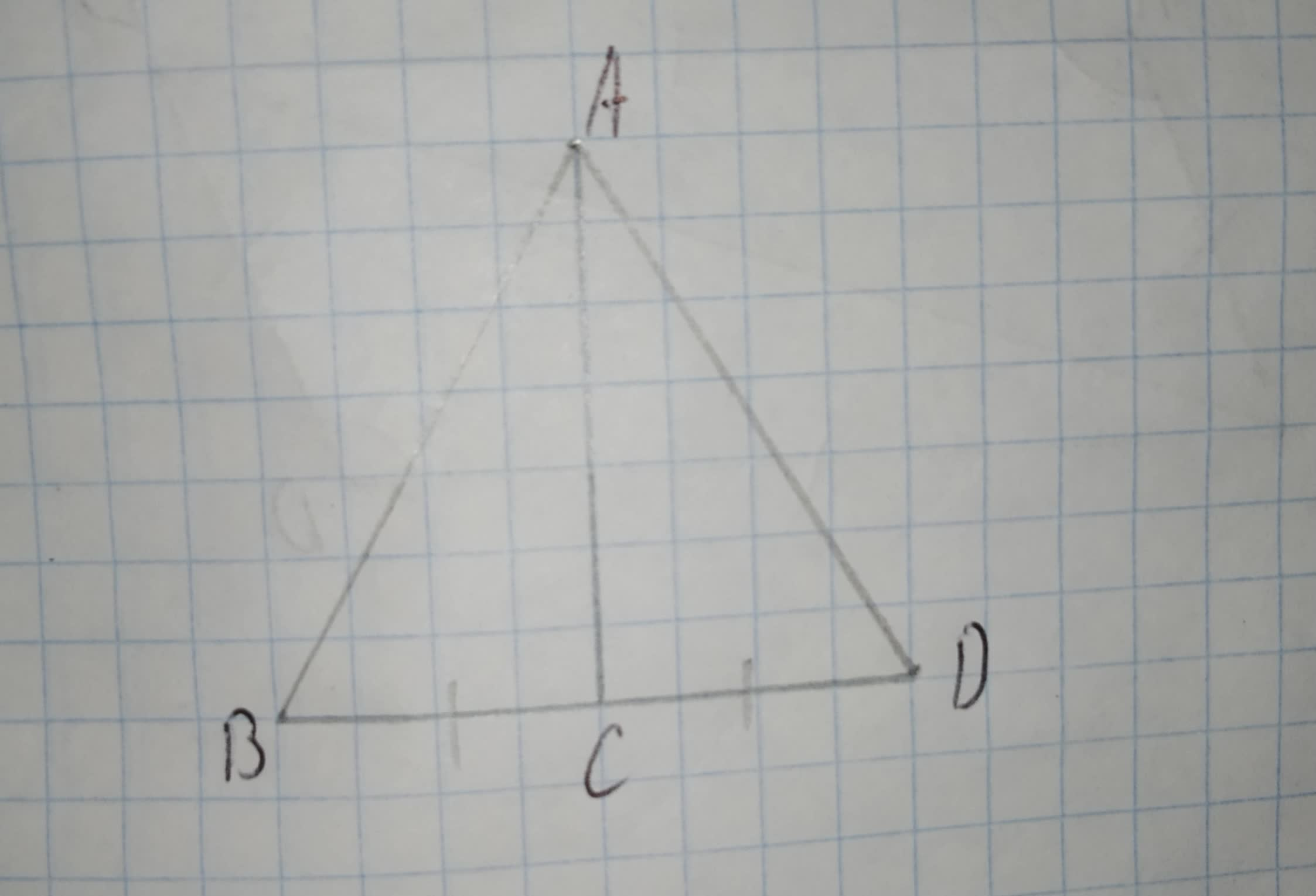# What other information do you need in order to prove the triangles congruent using the SAS Congruence Postulate?2210203782.jpgA)/_BAC ~=/_DACB)bar(AC) ~= bar(BD)C)/_BCA ~=/_DCAD)bar(AC) ~= bar(BD)bobbie71G 2021-03-09 Answered

What other information do you need in order to prove the triangles congruent using the SAS Congruence Postulate?A)$\mathrm{\angle }BAC\stackrel{\sim }{=}\mathrm{\angle }DAC$
B)$\stackrel{―}{AC}\stackrel{\sim }{=}\stackrel{―}{BD}$
C)$\mathrm{\angle }BCA\stackrel{\sim }{=}\mathrm{\angle }DCA$
D)$\stackrel{―}{AC}\stackrel{\sim }{=}\stackrel{―}{BD}$

You can still ask an expert for help

## Want to know more about Congruence?

• Questions are typically answered in as fast as 30 minutes

Solve your problem for the price of one coffee

• Math expert for every subject
• Pay only if we can solve it2abehn

Step 1
Given
The triangle areStep 2
To determine what information need to prove triangles congruent using SAS congruence postulate .
To prove SAS congruence postulate , We need to prove two sides and one angle are congregants in triangle $\mathrm{△}ACB\phantom{\rule{1em}{0ex}}\text{and}\phantom{\rule{1em}{0ex}}\mathrm{△}ACD$
By using given diagram
BC is congruent CD to prove $\mathrm{△}ACB\stackrel{\sim }{=}\mathrm{△}ACD$
AC is congruent to AC, Using reflective property .
In triangle ABC , AC is angle bisector of angle A and side bisector of BD
By using angle bisector theorem
$\mathrm{\angle }BAC\stackrel{\sim }{=}\mathrm{\angle }DAC$.
Therefore , To prove triangles congruent using SAS congruence postulate you need information $\mathrm{\angle }BAC\stackrel{\sim }{=}\mathrm{\angle }DAC$.